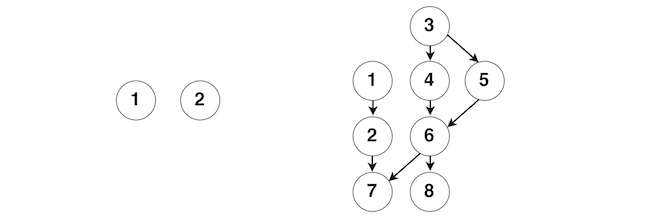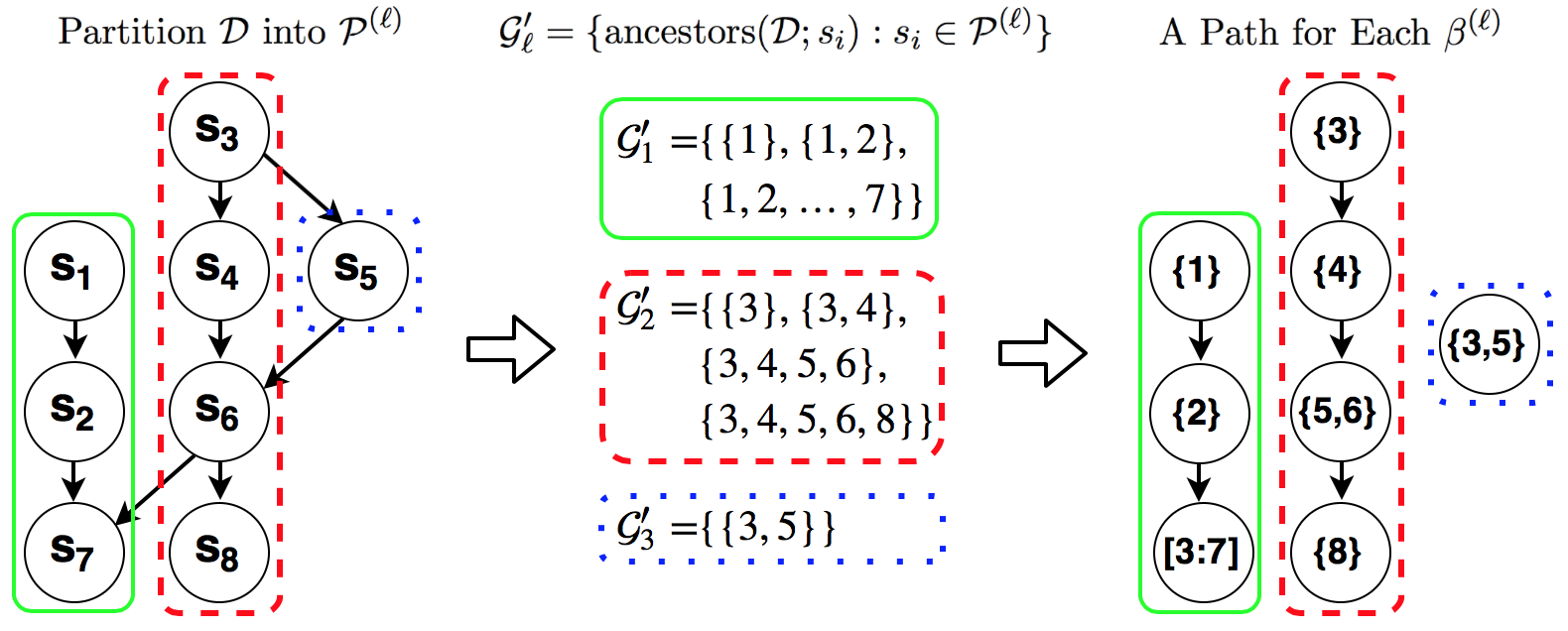# Using the hsm package

#### 2018-03-22

The hsm package implements an algorithm utilizing BCD blocked by path graphs for solving proximal operator of latent group Lasso in hierarchical sparse modeling, that is described in Yan and Bien (2017). This document introduces how to use this package.

## Knowing what problem is solved here

The solved problem is proximal operator of latent group lasso \begin{aligned} &\min_{\beta\in\mathbb R^p}\frac{1}{2}\left\|y - \beta \right\|_2^2 + \lambda *\Omega_{latent}^{\mathcal G}(\beta;w)\\ \text{where }&\Omega_{latent}^{\mathcal G}(\beta;w) = \min_{\substack{ supp(v^{(g)})\subset g, \text{ for }g\in\mathcal G\\\sum_{g\in\mathcal G}v^{(g)}=\beta}} w_g\left\|v^{(g)}\right\|_2 \end{aligned} for which the set of groups $$\mathcal G$$ are constructed so that a hierarchical sparsity pattern can be achieved in the solution. More specifically, for parameter of interest $$\beta$$, we define sets $$s_1, \dots, s_{n.nodes}\subset[1:p]$$ such that $$s_i\cap s_j=\emptyset$$ for $$i\neq j$$ and $$s_i\neq\emptyset$$ for all $$i\in[1:n.nodes]$$. A directed acyclic graph (DAG) defined on $$\{s_1,\dots,s_{n.nodes}\}$$ encodes the desired hierarchical structure in that when we set $$\beta_{s_i}$$ to zero, we have $\beta_{s_i}=0\quad\Rightarrow\quad\beta_{s_j}=0\quad\text{if }s_i\in ancestor(s_j)$ where $$ancestor(s_j)=\{s_k:s_k\text{ is in an ancestor node of }s_j,k\in[1:n.nodes]\}$$. The details of $$\mathcal G$$ can be found in Section 2.2 of Yan & Bien (2015).

In short, if you have a parameter vector $$\beta$$ embedded in a DAG and you desire the support to be parameters embedded in the a top portion of the DAG, solving the problem here can accomplish the goal of parameter selection. In addition, the solver can be used as a core step in a collection of proximal gradient methods including ISTA and FISTA. Please pay attention to the two requirements on DAG specified above:

• No two nodes shall share common parameters ($$s_i\cap s_j=\emptyset$$);
• There is no empty node in DAG ($$s_i\neq\emptyset$$).

Making sure the requirements satisfied are essential to the successful run of the package.

### Inputs for functions

There are two main functions in the package: hsm and hsm.path. The difference between them is that path solves the problem for a single $$\lambda$$ value, while hsm.path does that over a grid of $$\lambda$$ values. Besides the inputs for $$\lambda$$, these two functions share the same inputs. For the functions to work, you need to provide either map and var, or assign and w.assign. Generally, map and var are easier to define, and if provided they will be used to compute assign and w.assign. Meanwhile, the construction of assign and w.assign are related to the decomposition of DAG, which we will discuss in the next section. The following two examples show how to define map and var based on DAG and $$\beta$$.image

1. Example 1: The DAG contains two nodes on the left panel of the above figure. There are no directed edges connecting the two nodes. Suppose $$\beta\in\mathbb R^{10}$$ such that $$\beta_1$$ through $$\beta_{10}$$ are embedded in Node $$1$$ and the rest are embedded in Node $$2$$. The inputs we have for this DAG is as the following.
map <- matrix(c(1, NA, 2, NA), ncol = 2, byrow = TRUE)
map
##      [,1] [,2]
## [1,]    1   NA
## [2,]    2   NA
var <- list(1:10, 11:20)
var
## []
##    1  2  3  4  5  6  7  8  9 10
##
## []
##   11 12 13 14 15 16 17 18 19 20
1. Example 2: The DAG is on the right panel of the above figure. Suppose $$\beta\in\mathbb R^8$$ and $$\beta_i$$ is embedded in Node $$i$$ for $$i\in [1:8]$$. The inputs we have for this DAG is as the following.
map <- matrix(c(1, 2, 2, 7, 3, 4, 4, 6, 6, 7, 6, 8, 3, 5, 5, 6),
ncol = 2, byrow = TRUE)
map 
##      [,1] [,2]
## [1,]    1    2
## [2,]    2    7
## [3,]    3    4
## [4,]    4    6
## [5,]    6    7
## [6,]    6    8
## [7,]    3    5
## [8,]    5    6
var <- as.list(1:8)
var
## []
##  1
##
## []
##  2
##
## []
##  3
##
## []
##  4
##
## []
##  5
##
## []
##  6
##
## []
##  7
##
## []
##  8

Another argument requiring more attention is the weight vector for groups in $$\mathcal G$$. Since groups in $$\mathcal G$$ are one-one correspondence to the nodes in DAG, the elements in w should be of the same order as the indices for nodes. Under default value NULL for w, hsm and hsm.path will use $$w_l=\sqrt{\left|g_l\right|}$$ as the weight applied to group $$g_l$$. If you choose to pass in values for w yourself, you need to make sure $$w_l$$ increases with the size of $$g_l$$, i.e., if $$\left|g_{l_1}\right | > \left|g_{l_2}\right |$$, $$w_{l_1}> w_{l_2}$$; otherwise, the functions will fail.

## Descrbing how the package works

Prior to hsm, an intuitive and commonly-used way to solve the proximal operator is running BCD across groups in $$\mathcal G$$ as described in Section 4.1 of the paper. That approach can be computationally intensive, given the number of groups in $$\mathcal G$$ can be large and convergence is slow. Our path-based BCD takes advantage of the fact that the proximal operator can be exactly solved if all the parameters are embedded in a path graph, which is the finding in Section 4.2 of the paper. To work, hsm breaks down DAG into non-overlapping path graphs and runs BCD over parameters across the path graphs. For more details, see Section 4.2 and 4.3 of the paper. The number of path graphs is no larger than the number of nodes (in many cases, the difference is considerably large), which allows hsm to update more parameters per cycle and to enjoy better convergence rate.

### Breaking down DAG into path graphs

We use Example 2 to show how hsm breaks down a DAG. In the DAG of Example 2, there are two root nodes: Node 1 and Node 3. All the nodes in the DAG are descendants of at least one of the root nodes. At every root, hsm finds all possible path graphs originated from that root, picks up the one that consists of the most unmarked nodes, and marks the nodes in the selected path graphs. hsm will move to the next root only after all the descendant nodes of the current root have been marked. In Example 2, hsm is initially at Node 1. Since there is only one path graph consisting of nodes $$\{1, 2, 7\}$$ originated from 1, hsm selects it and mark nodes $$\{1,2,7\}$$. Then, hsm moves to the next root which is Node 3. There are four path graphs originated from Node 3: $$\{3,4,6,7\}$$, $$\{3,4,6,8\}$$, $$\{3,5,6,7\}$$ and $$\{3,5,6,8\}$$. Both $$\{3,4,6,8\}$$ and $$\{3,5,6,8\}$$ have 4 unmarked nodes. Under a tie situation, hsm picks up the first one and marks $$\{3,4,6,8\}$$. Now only one node is left unmarked, so Node 5 will be a path graph by itself.The above figure shows the decomposition of DAG in Example 2 (suppose $$s_i=\{i\}$$ for $$i=1, \cdots, 8$$ in the left panel). On the left panel, the three colored contours correspond to the three generated path graphs. Given we assume Node $$i$$ only contains parameter $$\beta_i$$ in this example, we are able to show the embedded parameters in the three path graphs in the right panel. The example is more throughly explained in Figure 7 of the paper. In the package, the function paths performs the decomposition of DAG and generates assign and w.assign.

library(hsm)
map <- matrix(c(1, 2, 2, 7, 3, 4, 4, 6, 6, 7, 6, 8, 3, 5, 5, 6),
ncol = 2, byrow = TRUE)
var <- as.list(1:8)
paths(map, var)
## $assign ## [,1] [,2] [,3] [,4] [,5] [,6] [,7] [,8] ## [1,] 1 2 3 3 3 3 3 0 ## [2,] 0 0 1 2 3 3 0 4 ## [3,] 0 0 1 0 1 0 0 0 ## ##$w.assign
## $w.assign[] ##  1.000000 1.414214 2.645751 ## ##$w.assign[]
##  1.000000 1.414214 2.000000 2.236068
##
## $w.assign[] ##  1.414214 ## Getting solutions along the solution path The functiont hsm takes the input y and returns a solution to the proximal operator, $$\hat\beta$$. The sparsity level of $$\hat\beta$$ depends on the magnitude of the tuning parameter $$\lambda$$. The function hsm.path gets $$\hat \beta$$ along a (log-linear) grid of $$\lambda$$ values. By default, a grid is used that starts at a value of $$\lambda$$ for which $$\hat\beta$$ is a zero vector. Users may supply this grid of $$\lambda$$ values using the argument lamlist. Following the specification in Example 2, the solutions along the solution path is library(hsm) map <- matrix(c(1, 2, 2, 7, 3, 4, 4, 6, 6, 7, 6, 8, 3, 5, 5, 6), ncol = 2, byrow = TRUE) var <- as.list(1:8) set.seed(100) y <- rnorm(8) result.path <- hsm.path(y=y, map=map, var=var, get.penalval=TRUE) Let’s look at $$\hat\beta$$ generated. round(result.path$beta.m, digits = 4)
##          [,1]   [,2]    [,3]   [,4]   [,5]   [,6]    [,7]   [,8]
##  [1,]  0.0000 0.0000  0.0000 0.0000 0.0000 0.0000  0.0000 0.0000
##  [2,] -0.0082 0.0000 -0.0170 0.1909 0.0000 0.0000  0.0000 0.0000
##  [3,] -0.1145 0.0000 -0.0303 0.3407 0.0177 0.0482  0.0000 0.1080
##  [4,] -0.1979 0.0064 -0.0408 0.4582 0.0412 0.1122 -0.0284 0.2356
##  [5,] -0.2634 0.0320 -0.0490 0.5505 0.0634 0.1728 -0.1414 0.3313
##  [6,] -0.3148 0.0527 -0.0554 0.6228 0.0782 0.2130 -0.2331 0.4101
##  [7,] -0.3552 0.0693 -0.0605 0.6797 0.0883 0.2406 -0.3066 0.4738
##  [8,] -0.3868 0.0825 -0.0645 0.7242 0.0955 0.2601 -0.3650 0.5246
##  [9,] -0.4116 0.0926 -0.0678 0.7618 0.1005 0.2737 -0.4096 0.5630
## [10,] -0.4311 0.1007 -0.0703 0.7903 0.1043 0.2840 -0.4454 0.5941
## [11,] -0.4464 0.1071 -0.0723 0.8121 0.1071 0.2918 -0.4739 0.6191
## [12,] -0.4584 0.1123 -0.0738 0.8288 0.1093 0.2978 -0.4967 0.6390
## [13,] -0.4678 0.1164 -0.0749 0.8417 0.1110 0.3024 -0.5147 0.6549
## [14,] -0.4752 0.1196 -0.0758 0.8516 0.1123 0.3060 -0.5290 0.6676
## [15,] -0.4810 0.1221 -0.0765 0.8593 0.1134 0.3088 -0.5402 0.6775
## [16,] -0.4856 0.1241 -0.0770 0.8653 0.1141 0.3109 -0.5491 0.6854
## [17,] -0.4892 0.1257 -0.0774 0.8700 0.1148 0.3126 -0.5561 0.6916
## [18,] -0.4920 0.1270 -0.0777 0.8737 0.1152 0.3139 -0.5616 0.6965
## [19,] -0.4942 0.1279 -0.0780 0.8765 0.1156 0.3149 -0.5659 0.7004
## [20,] -0.4959 0.1287 -0.0782 0.8787 0.1159 0.3157 -0.5693 0.7034

By adjusting flmin (which is the ratio between the largest and smallest $$\lambda$$ values in the grid) and nlam (which is the number of grid points used), you can get your desired sparsity level in the solutions.